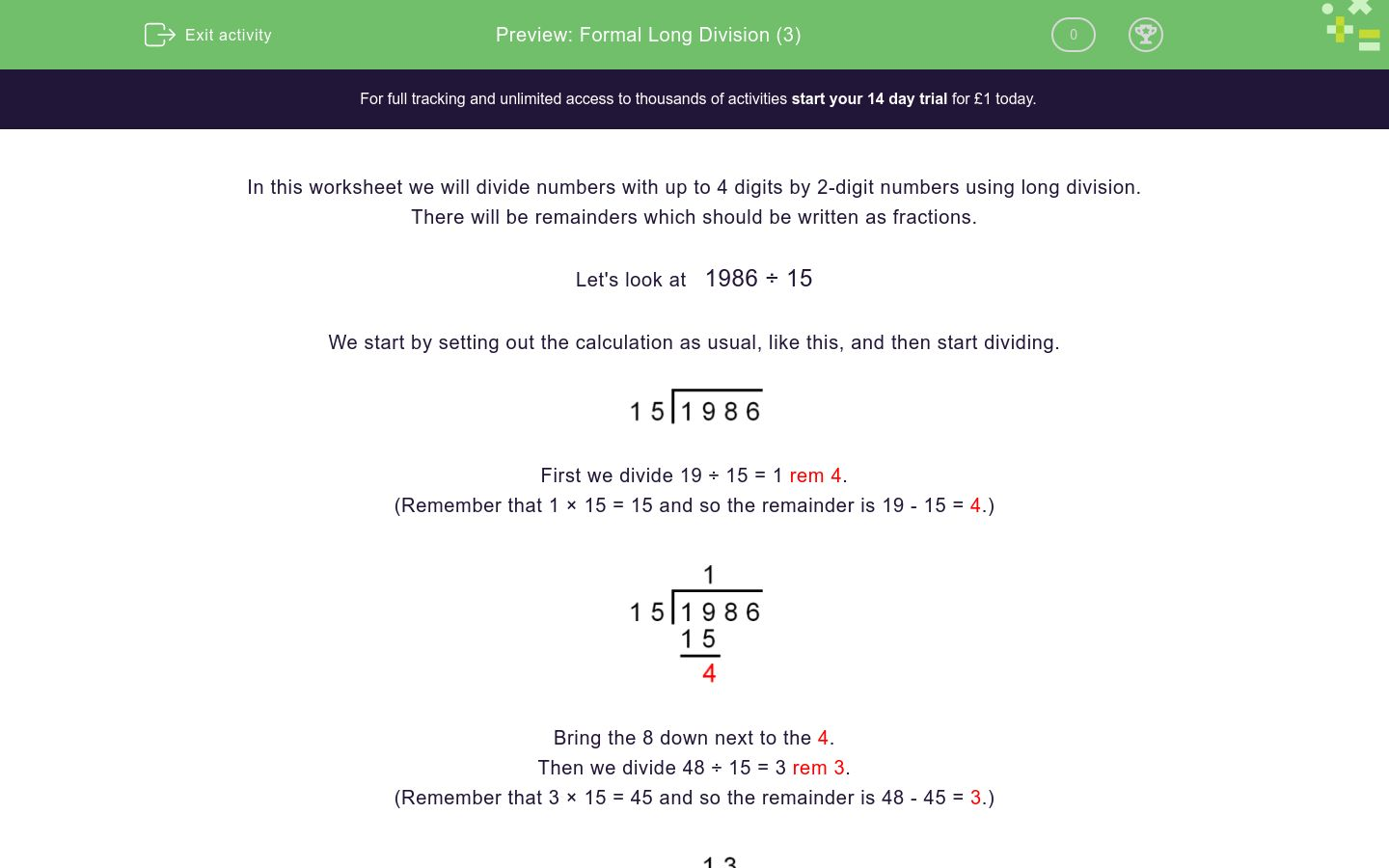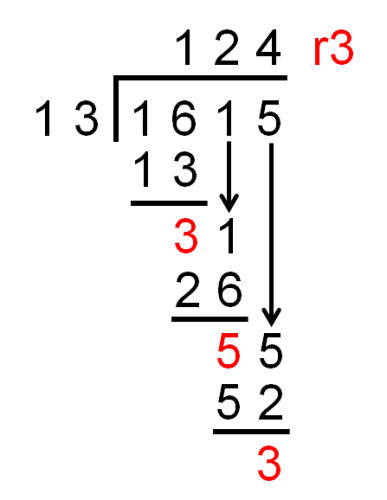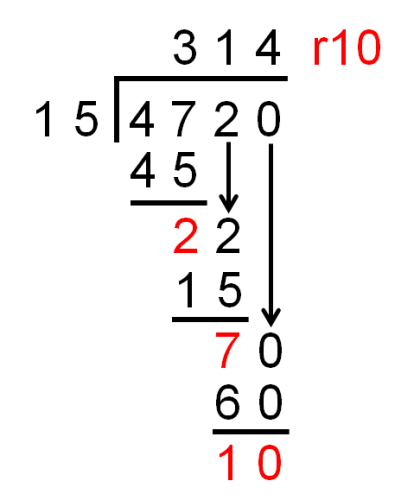# Formal Long Division (3)

In this worksheet, students must divide numbers up to 4 digits by a two-digit number using the formal written method of long division. There may be remainders, which should be written as reduced fractions.Key stage:  KS 2

Curriculum topic:  Number: Addition, Subtraction, Multiplication and Division

Curriculum subtopic:  Divide to Four Digits (Long Division)

Difficulty level:### QUESTION 1 of 10

In this worksheet we will divide numbers with up to 4 digits by 2-digit numbers using long division.

There will be remainders which should be written as fractions.

Let's look at   1986 ÷ 15

We start by setting out the calculation as usual, like this, and then start dividing.First we divide 19 ÷ 15 = 1 rem 4.

(Remember that 1 × 15 = 15 and so the remainder is 19 - 15 = 4.)Bring the 8 down next to the 4.

Then we divide 48 ÷ 15 = 3 rem 3.

(Remember that 3 × 15 = 45 and so the remainder is 48 - 45 = 3.)Bring the 6 down next to the 3.

Now we divide 36 ÷ 15 = 2 rem 6.

(Remember that 2 × 15 = 30 and so the remainder is 39 - 30 = 9.)The final remainder is 6, but we want to write this as a fraction.

We are dividing by 15, so a remainder of 6 is actually the fraction 6/15.

 6 15

To reduce this fraction to its lowest terms, we look for the highest number that goes into 6 and 15.

This will be 3, because 2 × 3 = 6 and 5 × 3 = 15.

So we divide the top and the bottom numbers by the magic number 3, to get 2 and 5.

 6 = 2 15 5

We have shown that 1986 ÷ 15 =

 132 2 5

In this worksheet, this will be written as 132 2/5  with a space between the 132 and the 2/5.

On paper, use long division to work out the answer to:

1615 ÷ 13

(Write any remainder as a fraction, e.g. 343 3/4 with one space between the whole number and the fraction.)

On paper, use long division to work out the answer to:

3904 ÷ 12

(Write any remainder as a reduced fraction, e.g. 343 3/4 with one space between the whole number and the fraction.)

On paper, use long division to work out the answer to:

3608 ÷ 15

(Write any remainder as a reduced fraction, e.g. 343 3/4 with one space between the whole number and the fraction.)

On paper, use long division to work out the answer to:

3396 ÷ 16

(Write any remainder as a reduced fraction, e.g. 343 3/4 with one space between the whole number and the fraction.)

On paper, use long division to work out the answer to:

5195 ÷ 11

(Write any remainder as a reduced fraction, e.g. 343 3/4 with one space between the whole number and the fraction.)

On paper, use long division to work out the answer to:

4154 ÷ 12

(Write any remainder as a reduced fraction, e.g. 343 3/4 with one space between the whole number and the fraction.)

On paper, use long division to work out the answer to:

9858 ÷ 12

(Write any remainder as a reduced fraction, e.g. 343 3/4 with one space between the whole number and the fraction.)

On paper, use long division to work out the answer to:

3101 ÷ 14

(Write any remainder as a reduced fraction, e.g. 343 3/4 with one space between the whole number and the fraction.)

On paper, use long division to work out the answer to:

4720 ÷ 15

(Write any remainder as a reduced fraction, e.g. 343 3/4 with one space between the whole number and the fraction.)

On paper, use long division to work out the answer to:

5535 ÷ 25

(Write any remainder as a reduced fraction, e.g. 343 3/4 with one space between the whole number and the fraction.)

• Question 1

On paper, use long division to work out the answer to:

1615 ÷ 13

(Write any remainder as a fraction, e.g. 343 3/4 with one space between the whole number and the fraction.)

124 3/13
EDDIE SAYS• Question 2

On paper, use long division to work out the answer to:

3904 ÷ 12

(Write any remainder as a reduced fraction, e.g. 343 3/4 with one space between the whole number and the fraction.)

325 1/3
EDDIE SAYS4/12 reduces to 1/3.
• Question 3

On paper, use long division to work out the answer to:

3608 ÷ 15

(Write any remainder as a reduced fraction, e.g. 343 3/4 with one space between the whole number and the fraction.)

240 8/15
EDDIE SAYS8/15 does not reduce.
• Question 4

On paper, use long division to work out the answer to:

3396 ÷ 16

(Write any remainder as a reduced fraction, e.g. 343 3/4 with one space between the whole number and the fraction.)

212 1/4
EDDIE SAYS4/16 reduces to 1/4.
• Question 5

On paper, use long division to work out the answer to:

5195 ÷ 11

(Write any remainder as a reduced fraction, e.g. 343 3/4 with one space between the whole number and the fraction.)

472 3/11
EDDIE SAYS3/11 does not reduce.
• Question 6

On paper, use long division to work out the answer to:

4154 ÷ 12

(Write any remainder as a reduced fraction, e.g. 343 3/4 with one space between the whole number and the fraction.)

346 1/6
EDDIE SAYS2/12 reduces to 1/6.
• Question 7

On paper, use long division to work out the answer to:

9858 ÷ 12

(Write any remainder as a reduced fraction, e.g. 343 3/4 with one space between the whole number and the fraction.)

821 1/2
EDDIE SAYS6/12 reduces to 1/2.
• Question 8

On paper, use long division to work out the answer to:

3101 ÷ 14

(Write any remainder as a reduced fraction, e.g. 343 3/4 with one space between the whole number and the fraction.)

221 1/2
EDDIE SAYS7/14 reduces to 1/2.
• Question 9

On paper, use long division to work out the answer to:

4720 ÷ 15

(Write any remainder as a reduced fraction, e.g. 343 3/4 with one space between the whole number and the fraction.)

314 2/3
EDDIE SAYS10/15 reduces to 2/3.
• Question 10

On paper, use long division to work out the answer to:

5535 ÷ 25

(Write any remainder as a reduced fraction, e.g. 343 3/4 with one space between the whole number and the fraction.)

221 2/5
EDDIE SAYS10/25 reduces to 2/5.
---- OR ----

Sign up for a £1 trial so you can track and measure your child's progress on this activity.

### What is EdPlace?

We're your National Curriculum aligned online education content provider helping each child succeed in English, maths and science from year 1 to GCSE. With an EdPlace account you’ll be able to track and measure progress, helping each child achieve their best. We build confidence and attainment by personalising each child’s learning at a level that suits them.